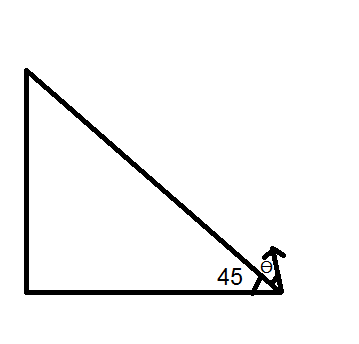Projectile on a inclined planeA particle is projected from the bottom of a inclined plane at an angle $\theta$ with the surface of incline. The incline has an inclination of 1 i.e. angle of incline is 45 degrees. For what value of $\theta$ will the particle retrace its path after collision with the plane.

*Note *

• the collision is an elastic collision.
• the answer is of the form $\theta$ = $cot^{-1}x$ Find $x$
×